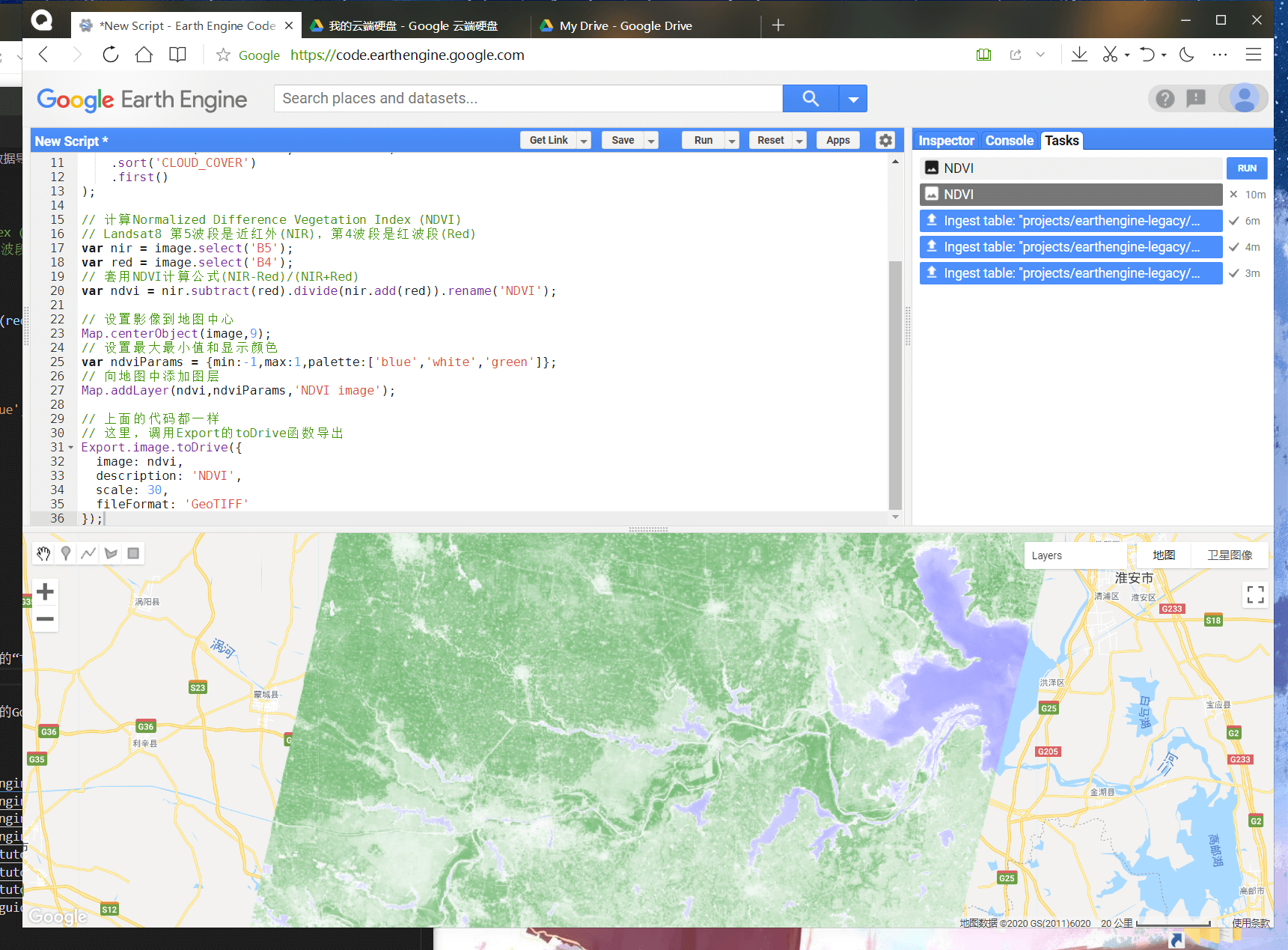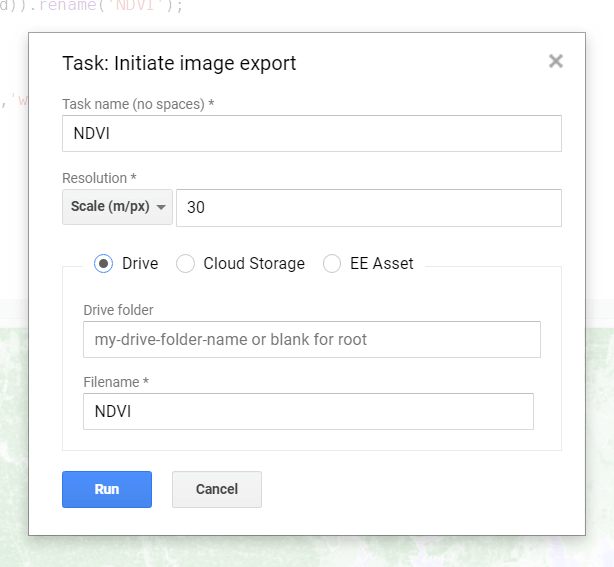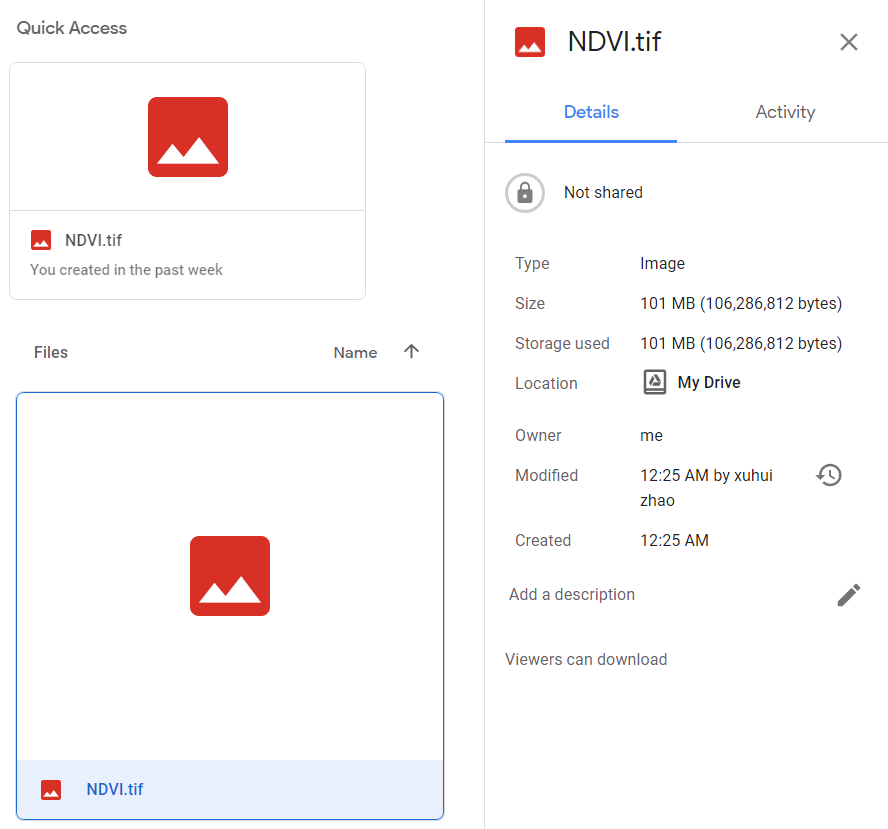## Oct 14,2020   10407 words   38 min

#### 2.基本数据类型

``````// 新建一个服务器上的字符串
var serverString = ee.String('This is on the server.');
print('String on the server:', serverString);

// 新建一个服务器上的数字
var serverNumber = ee.Number(3.14159);
print('number=',serverNumber);

// 新建一个服务器上的列表
var serverList = ee.List([1,5,6,9,2]);
print('Sequence:',serverList);
// 注意，由于是服务器上的List，所以需要通过get()函数获得内容，而不是直接索引
print('item 2:',serverList.get(2));

// 新建一个服务器上的字典，包含三个键值对
var serverDict = ee.Dictionary({
e:Math.E,
pi:Math.PI,
phi:(1+Math.sqrt(5))/2
});
// 和上面一样，需要通过get()函数获取数据
print('Golden ratio:',serverDict.get('phi'));
print('Keys:',serverDict.keys());

// 新建一个服务器上的日期
var serverDate = ee.Date('2020-10-13');
print('date:',serverDate);
``````

#### 3.基本语法

##### (1)循环

``````// This generates a list of numbers from 1 to 10.
var myList = ee.List.sequence(1, 10);

// The map() operation takes a function that works on each element independently
// and returns a value. You define a function that can be applied to the input.
var computeSquares = function(number) {
// We define the operation using the EE API.
return ee.Number(number).pow(2);
};

// Apply your function to each item in the list by using the map() function.
var squares = myList.map(computeSquares);
print(squares);  // [1, 4, 9, 16, 25, 36, 49, 64, 81]
``````
##### (2)条件判断

``````// The following function determines if a number is even or odd.  The mod(2)
// function returns 0 if the number is even and 1 if it is odd (the remainder
// after dividing by 2).  The input is multipled by this remainder so even
// numbers get set to 0 and odd numbers are left unchanged.
var getOddNumbers = function(number) {
number = ee.Number(number);   // Cast the input to a Number so we can use mod.
var remainder = number.mod(2);
return number.multiply(remainder);
};

// This generates a list of numbers from 1 to 10.
var myList = ee.List.sequence(1, 10);

// filtering
var newList = myList.map(getOddNumbers);

// Remove the 0 values.
var oddNumbers = newList.removeAll();
print(oddNumbers);
``````

``````var collection = ee.ImageCollection('LANDSAT/LC08/C01/T1_TOA');

// Divide the collection into 2 subsets and apply a different algorithm on them.
var subset1 = collection.filter(ee.Filter.lt('SUN_ELEVATION', 40));
var subset2 = collection.filter(ee.Filter.gte('SUN_ELEVATION', 40));

var processed1 = subset1.map(function(image) {
return image.multiply(2);
});
var processed2 = subset2;

// Merge the collections to get a single collection.
var final = processed1.merge(processed2);
print('Original collection size', collection.size());
print('Processed collection size', final.size());
``````

##### (3)累加

``````var algorithm = function(current, previous) {
previous = ee.List(previous);
var n1 = ee.Number(previous.get(-1));
var n2 = ee.Number(previous.get(-2));
};

// Compute 10 iterations.
var numIteration = ee.List.repeat(1, 10);
var start = [0, 1];
var sequence = numIteration.iterate(algorithm, start);
print(sequence);  // [0, 1, 1, 2, 3, 5, 8, 13, 21, 34, 55, 89]
``````

#### 4.可视化影像与波段

``````// Instantiate an image with the Image constructor.
var image = ee.Image('CGIAR/SRTM90_V4');

// Zoom to a location.
Map.setCenter(117.3798, 32.9049, 4); // Center on the Bengbu City.

// Display the image on the map.
``````

#### 5.影像集

``````//  获取Landsat8 TOA影像
var l8 = ee.ImageCollection('LANDSAT/LC08/C01/T1_TOA');

// 定义一个目标点
var point = ee.Geometry.Point(117.0721,33.2363);

// 调用filterBounds筛选位置
var spatialFiltered = l8.filterBounds(point);
print('spatialFiltered',spatialFiltered);

// 再调用filterDate筛选时间
var temporalFiltered = spatialFiltered.filterDate('2015-01-01','2015-12-31');
print('temporalFiltered',temporalFiltered);

// 到这一步之后，还可能有很多影像的，我们可以进一步按照云层覆盖进行排序
var sorted = temporalFiltered.sort('CLOUD_COVER');
// 选取含云量最少的那一景
var scene = sorted.first();

// 将影像放到当前地图的中心，缩放9级
Map.centerObject(scene,9);

// Landsat8的RGB波段分别是B4、B3、B2
// 最大值设为0.3，最小值默认为0
var visParams = {bands:['B4','B3','B2'],max:0.3};

// 展示影像，名称为true-color composite
``````

#### 6.实例练习-基于Landsat数据计算NDVI

``````// 指定某个目标点
var point = ee.Geometry.Point([117.0721,33.2363]);

// 导入Landsat-8 TOA影像数据集
var l8 = ee.ImageCollection('LANDSAT/LC08/C01/T1_TOA');

// 获取2015年云量最少的影像
var image = ee.Image(
l8.filterBounds(point)
.filterDate('2015-01-01', '2015-12-31')
.sort('CLOUD_COVER')
.first()
);

// 计算Normalized Difference Vegetation Index (NDVI)
// Landsat8 第5波段是近红外(NIR)，第4波段是红波段(Red)
var nir = image.select('B5');
var red = image.select('B4');
// 套用NDVI计算公式(NIR-Red)/(NIR+Red)

// 设置影像到地图中心
Map.centerObject(image,9);
// 设置最大最小值和显示颜色
var ndviParams = {min:-1,max:1,palette:['blue','white','green']};
// 向地图中添加图层
``````

``````// 导入Landsat-8 TOA影像数据集
var l8 = ee.ImageCollection('LANDSAT/LC08/C01/T1_TOA');

var ndvi = image.normalizedDifference(['B5', 'B4']).rename('NDVI');
};

// 利用GEE的map函数并行计算NDVI

// 我们可用计算好的NDVI找到所有影像中最“绿”的像素
var greenest = withNDVI.qualityMosaic('NDVI');

// 展示结果
var visParams = {bands: ['B4', 'B3', 'B2'], max: 0.3};
``````

#### 7.数据导出

GEE虽然是云端处理，但显然是支持数据的导出和下载的，不然运行的结果没法使用。数据的导出主要分为两类，一类是运算的数值类型数据的导出以及绘图，一类是影像的导出。下面分别介绍。

##### (1)数值与绘图

``````// Import the Landsat 8 TOA image collection.
var l8 = ee.ImageCollection('LANDSAT/LC08/C01/T1_TOA');

// Map a function over the Landsat 8 TOA collection to add an NDVI band.
var withNDVI = l8.map(function(image) {
// Get a cloud score in [0, 100].
var cloud = ee.Algorithms.Landsat.simpleCloudScore(image).select('cloud');

// Create a mask of cloudy pixels from an arbitrary threshold.

// Compute NDVI.
var ndvi = image.normalizedDifference(['B5', 'B4']).rename('NDVI');

// Return the masked image with an NDVI band.
});

// Define ROI.
var roi = ee.Geometry.Polygon([[117.211,32.847],
[117.464,32.847],
[117.464,32.995],
[117.211,32.995],
[117.211,32.847]]);

// Create a chart.
var chart = ui.Chart.image.series({
imageCollection: withNDVI.select('NDVI'),
region: roi,
reducer: ee.Reducer.first(),
scale: 30
}).setOptions({title: 'NDVI over time'});

// Display the chart in the console.
print(chart);
Map.centerObject(roi,11);
``````

##### (2)保存影像

``````// 指定某个目标点
var point = ee.Geometry.Point([117.0721,33.2363]);

// 导入Landsat-8 TOA影像数据集
var l8 = ee.ImageCollection('LANDSAT/LC08/C01/T1_TOA');

// 获取2015年云量最少的影像
var image = ee.Image(
l8.filterBounds(point)
.filterDate('2015-01-01', '2015-12-31')
.sort('CLOUD_COVER')
.first()
);

// 计算Normalized Difference Vegetation Index (NDVI)
// Landsat8 第5波段是近红外(NIR)，第4波段是红波段(Red)
var nir = image.select('B5');
var red = image.select('B4');
// 套用NDVI计算公式(NIR-Red)/(NIR+Red)

// 设置影像到地图中心
Map.centerObject(image,9);
// 设置最大最小值和显示颜色
var ndviParams = {min:-1,max:1,palette:['blue','white','green']};
// 向地图中添加图层

// 上面的代码都一样
// 这里，调用Export的toDrive函数导出
Export.image.toDrive({
image: ndvi,
description: 'NDVI',
scale: 30
});
``````运行代码以后，除了会得到之前的结果外，在右上角的“Tasks”里也会看到有新的任务，点击“Run”，弹出如下对话框。填入相关信息，即可开始导出，导出完成后就会在你的Google Drive里看到对应的文件了，下载即可。如下图所示。